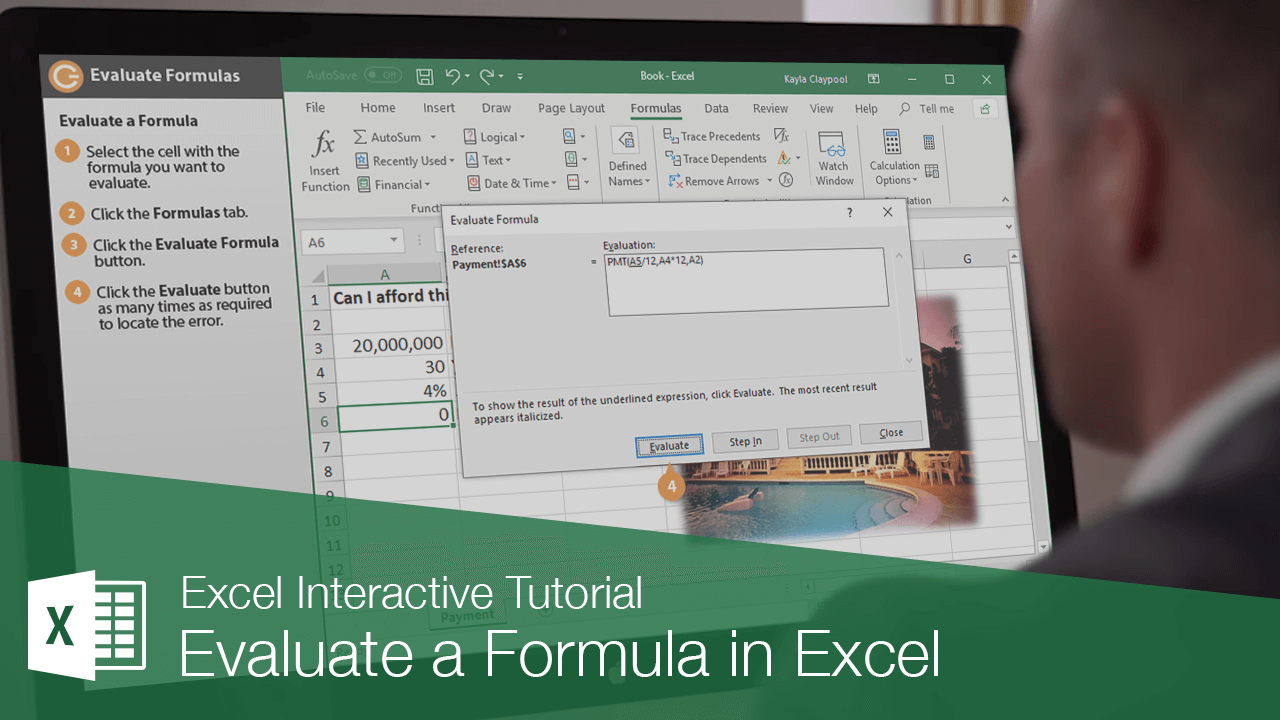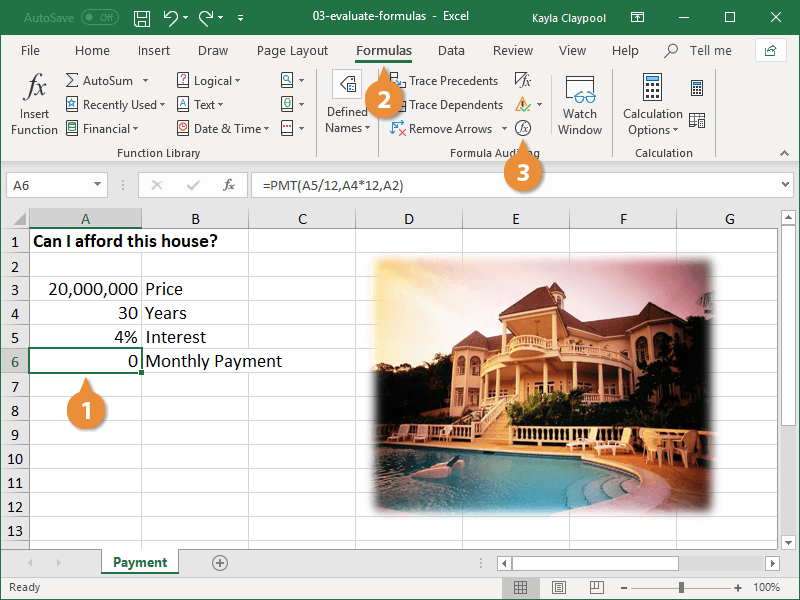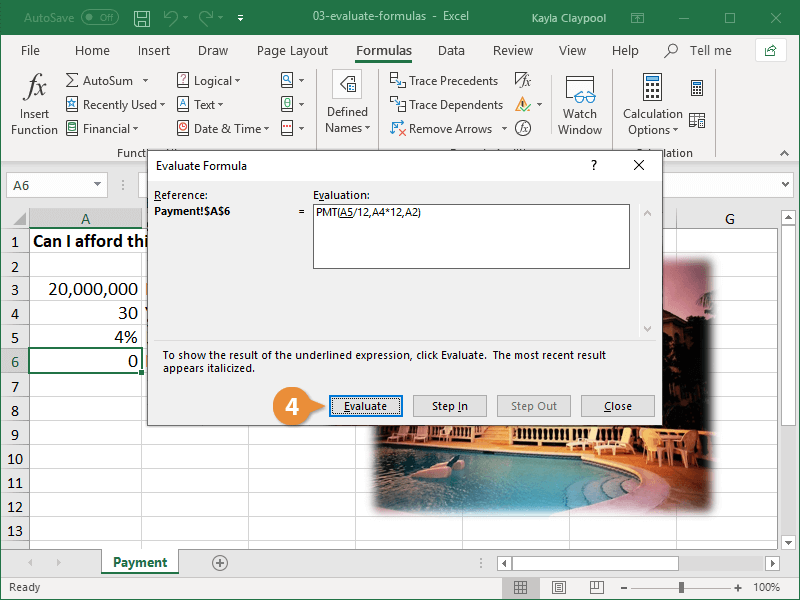Evaluate a Formula in Excel | CustomGuide# Evaluate a Formula in Excel

How to Evaluate Formulas in ExcelThe Evaluate Formula feature walks you through each argument in a formula to help identify and fix any mistakes. You can also use it to understand complex formulas, seeing how each part of a nested function is calculated to reach the final result.

### Evaluate a Formula

1. Select the cell with the formula you want to evaluate.
2. Click the Formulas tab.
3. Click the Evaluate Formulas button.The Evaluate Formula dialog box displays, which breaks down the formula arguments for you so that you can isolate the error.

With each click of the Evaluate button, Excel looks at the value of the underlined reference and returns the result, thus letting you analyze each component of the formula individually.

4. Click the Evaluate button as many times as required to locate the error.5. Click the Close button.
6. Fix any errors in the fomula.